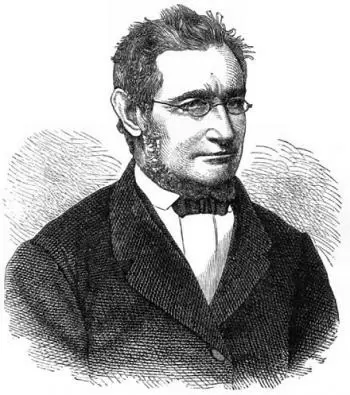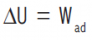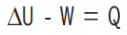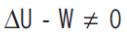# First Law of ThermodynamicsThe first law of thermodynamics states: "The total energy of an isolated system is neither created nor destroyed, it remains constant.” It is a principle that reflects the conservation of energy.

Energy cannot be created. It is only transformed from one type to another. When one class of energy disappears, an equivalent amount of another kind must be produced.

A body can have a certain speed, which involves kinetic energy. If the body loses momentum, it loses kinetic energy, transforming into another type of energy. The conversion can be to potential energy (if it acquires height), heat energy (if there is any type of friction that causes it to heat up), etc.

The first principle of thermodynamics allows us to define the postulate of the first law:

If we supply any adiabatic system with a certain amount of mechanical energy W, this energy only causes an increase in the internal energy of system U. So:It is the 1st of the fundamental laws of thermodynamics. However, later, the zeroth law of thermodynamics was added to the list.

## The First Law of Thermodynamics for Non-isolated Systems

If the system is not isolated, this equality is not fulfilled, and the system changes from heat.There is no trivial step of physical conception from the closed system view to an open system view for thermodynamics' first law.

For closed systems, the concepts of an adiabatic enclosure and an adiabatic wall are essential. Matter and internal energy cannot penetrate or penetrate such a wall. For a non-isolated system, there is a wall that allows the penetration of matter.

In general, matter in diffusive motion carries with it some internal energy. Some changes in microscopic potential energy accompany the movement. An open system is not adiabatically closed.

There are some cases where a thermodynamic process for a non-isolated system can, for particular purposes, be considered as an isolated system.

By definition, hypothetically or potentially, the matter can pass between the system and its environment in an open system. But when the process of interest involves only hypothetical or potential but not the actual passage of matter, the process can be considered as if it were for a closed system.

### Sign Criteria

In the case that the system does the work, W is negative. If the system does the work, the work done by the system is positive.

ΔQ is the amount of heat absorbed or emitted by a heat engine. If the net heat transfer is to the system, ΔQ will be positive. If the net energy transfer leaves the system, ΔQ will be negative.

## What Is an Adiabatic System?

An adiabatic process is a process in which the system does not exchange heat with its environment. It is in thermal equilibrium. An adiabatic process that is also reversible is isentropic.

The term adiabatic refers to volumes that prevent heat transfer with the environment. An isolated wall is quite close to an adiabatic limit.

An adiabatic process is performed with constant heat variation. In an isobaric process, it is carried out at constant pressure.

For all adiabatic processes that take a system from a given initial state to a given final state, the respective eventual total quantities of energy transferred as heat and work are the same. It is determined just by the given initial and final conditions.

## What Is Internal Energy?

Internal energy is the energy required to create a system in the absence of temperature or volume changes. It is a thermodynamic property—a state variable.

Joule postulate that:

If we supply any isolated thermodynamic system with a certain amount of mechanical energy W, it only causes an increase in the internal energy of system U:The internal energy variation is equal to the supplied work.

This equality that applies to the isolated system constitutes the definition of the internal energy U.

The internal energy in the international system of units is measured in joules (J).

The existence of this quantity for any system is the postulate known as the first principle of thermodynamics.

## What if the System Is Not Isolated?

If the system is not isolated, we observe that:The missing energy is due to thermal energy loss. Losses are expected to heat transfer from the system to the outside due to their temperature differences.

Then we can write:Author:

Published: July 1, 2016
Last review: October 29, 2020